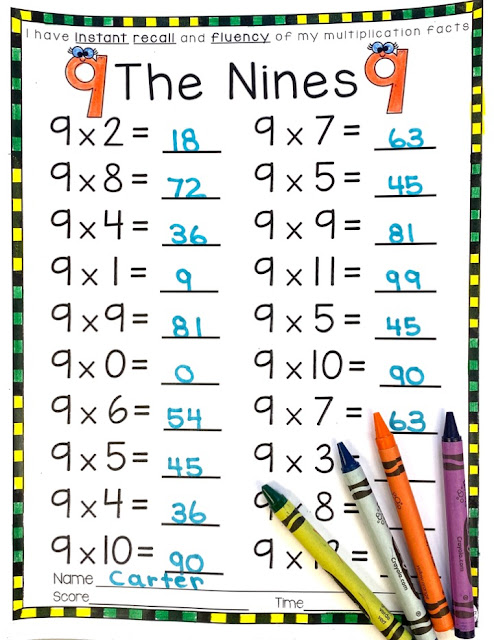## March 11, 2020

### 3 Cool + Simple Tricks to Multiply by 9 that your students will LOVE!

It's MULTIPLICATION TIME!  My students are so excited to be practicing their Nines Multiplication Facts now! Time to bring out the multiplication games and multiplication math centers.

How I introduce the 9 Times Tables

9s are super fun because there are so many great patterns and tricks when it comes to the Nines!  The first 9s trick that I love is how the product of 9 times any number always equals nine when you add the digits together.  I love watching my students' faces when I write the Multiplication Nines Facts on the Smartboard and we work together to prove it!

You can do this with literally any number:Multiply 9 by any number!

Another cool trick when you multiply by nine is that we notice a pattern with the first five facts (9x1=9 to 9x5=45) to the next five facts (9x6=54 to 9x10=90). The numbers in the product are reversed. 😉

I saved the best for last!  A third awesome trick (that I would have loved as a kid) is how you can use your fingers to help you multiply by 9.  First, hold out your hands and imagine that they are numbered 1-10, like this:

Then, if you are multiplying 9x4, you put down your fourth finger.  The fingers to the left of the fourth finger are the amount of tens, and the numbers to the right of the finger are the amount of ones!

Before and after I introduce a new multiplication family, I always begin with a pretest to see what my students know.  This helps me identify which students will need more support and additional time in my math small groups.  I use this worksheet below as a pre and post test. I'm offering it below to you as a freebie.Grab this freebie HERE
Once we understand multiplying by 9 using arrays, pictures and manipulatives, we begin practicing facts for accuracy and fluency.  There are several multiplication activities that the students enjoy including tic tac toe games, BUMP, Number Puzzles and Sorting Worksheets.

Here is one of our favorite practice activities. Cut, Sort, Glue.

In this multiplication practice activity, students cut our math facts and then decide if the fact is true or false.  Some students are able to do this independently while others need to draw pictures or make an array to get the multiplication product.  Either way, the kids enjoy practicing facts with this worksheet.

Grab this Multiplication Times 9 FREEBIE HERE